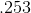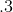SSAT Elementary Level Math : How to find the decimal equivalent of a fraction

Example Questions

Example Question #21 : How To Find The Decimal Equivalent Of A Fraction

What is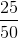equal to in decimal form?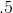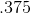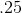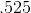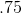Explanation:

To find the decimal equivalent of a fraction, divide the top number (numerator) by the bottom number (denominator). In this problem, 25 divided by 50 equals .5!

Example Question #22 : How To Find The Decimal Equivalent Of A Fraction

Rounded to the nearest tenth, what isequal to in decimal form?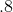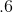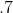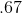Explanation:

To find the decimal equivalent of a fraction, divide the top number (numerator) by the bottom number (denominator). In this problem, 2 divided by 3 equals .667. But, the question asks us to round our answer to the nearest tenth, making the correct answer .7!

Example Question #7 : Other Fraction Manipulations

What is the decimal equivalent to?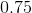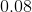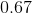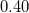Explanation:

The correct answer is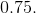is greater than half so you can eliminateandright away.

You should memorize common fractions: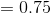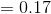Example Question #23 : How To Find The Decimal Equivalent Of A Fraction

What is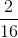in decimal form?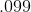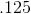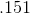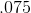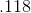Explanation:

To find the decimal equivalent of a fraction, simply divide the top number (numerator) by the bottom number (denominator). In this problem, 2 divided by 12 equals .125.

Example Question #24 : How To Find The Decimal Equivalent Of A Fraction

What is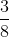in decimal form?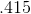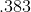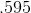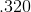Explanation:

To find the decimal equivalent of a fraction, simply divide the top number (numerator) by the bottom number (denominator). In this problem, 3 divided by 8 equals .375.

Example Question #25 : How To Find The Decimal Equivalent Of A Fraction

What isequal to in decimal form?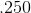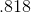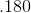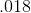Explanation:

To find the decimal equivalent of a fraction, simply divide the top number of the fraction (numerator) by the bottom number of the fraction (denominator). In this problem, 1 divided by 8 equals .125!

Example Question #26 : How To Find The Decimal Equivalent Of A Fraction

What is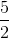equal to in decimal form?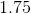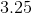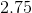Explanation:

To find the decimal equivalent of a fraction, simply divide the top number of the fraction (numerator) by the bottom number of the fraction (denominator). In this problem, 5 divided by 2 equals 2.5!

Example Question #27 : How To Find The Decimal Equivalent Of A Fraction

What is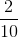equal to in decimal form?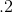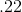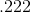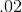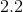Explanation:

To find the decimal equivalent of a fraction, divide the top number (numerator) by the bottom number (denominator). In this problem, 2 divided by 10 equals .2!

Example Question #28 : How To Find The Decimal Equivalent Of A Fraction

What isequal to in decimal form?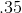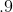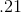Explanation:

To find the decimal equivalent of a fraction, divide the top number (numerator) by the bottom number (denominator). In this problem, 3 divided by 5 equals .6!

Example Question #29 : How To Find The Decimal Equivalent Of A Fraction

What isequal to in decimal form?